# Die Casting Process - The Force Factor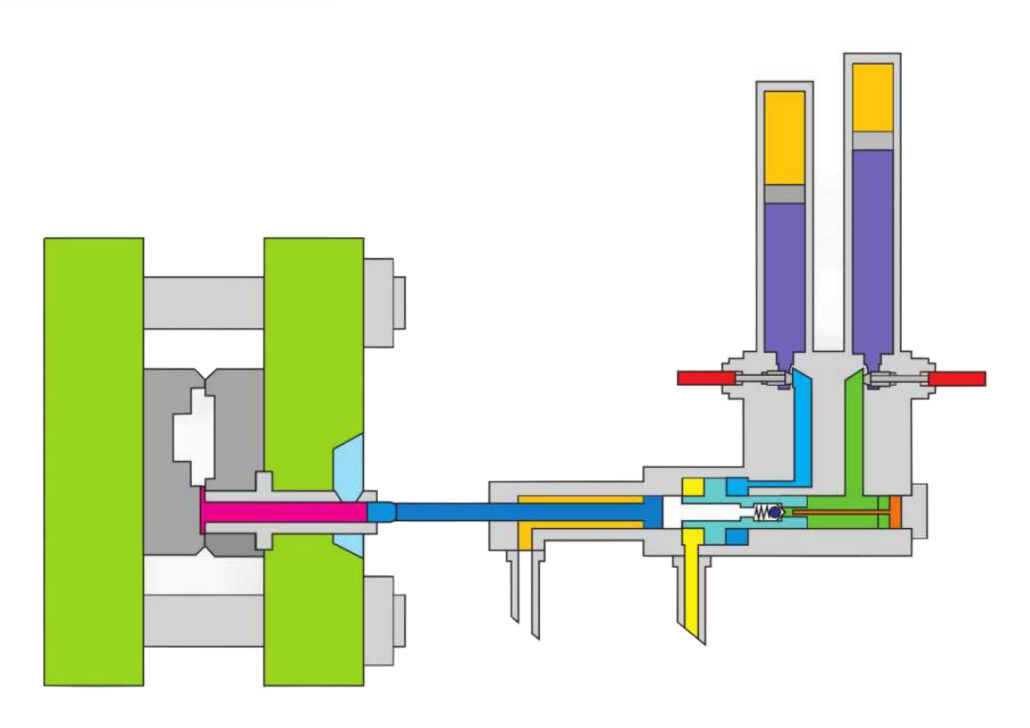It’s been a worthwhile journey, and now it’s time we study the die casting process. Of course, there is no die casting without its process, hence the need for this blog.

## 1.Features of Die Casting

Die casting, also known as pressure casting, is a casting method in which molten metal fills the mold cavity at high speed under high pressure and solidifies under pressure. Die casting has the characteristics of high pressure, high speed, and high temperature.

#### High pressure

The molten metal fills the mold cavity at very high pressures and crystallizes and solidifies at higher pressures. The common pressure is 15~500Mpa, which is equivalent to 5000 atmospheres.

The deepest trench on our planet is the Mariana Trench, which is 11,000 meters deep and has a pressure of 1,100 atmospheres. Therefore, the pressure exerted on the molten metal during die casting is equivalent to the pressure of 5 Mariana Trench.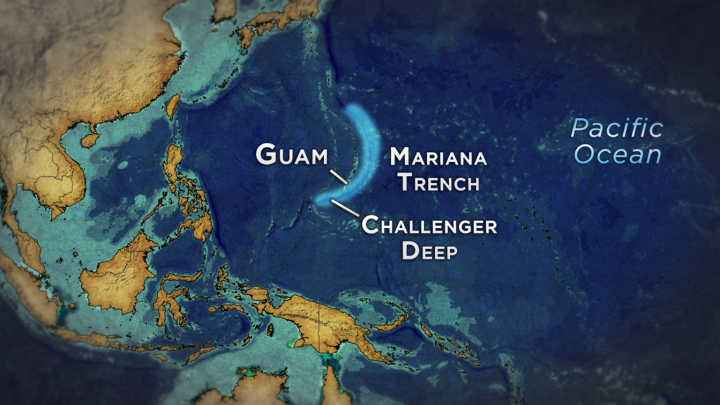#### High speed

The molten metal fills the cavity at high speed, usually 10-50 m/s, and some can exceed 100 m/s. Therefore, the filling time of the molten metal is very short, and the cavity can be filled in about 0.01-0.2 seconds (depending on the size of the casting).

#### High temperature

The temperature is subject to the different alloys that feature in the process.

• The pouring temperature of zinc alloy is: 400~420 ℃
• The pouring temperature of aluminum alloy is: 620~680 ℃
• The pouring temperature of magnesium alloy is: 640~690 ℃
• The pouring temperature of copper alloy is: 900~1100 ℃

## 2.Die Casting Process Parameters

The die-casting process is a process in which the three elements of the die-casting machine, die-casting mold, and die-casting alloy are organically combined and used comprehensively. The die casting production process’s main parameters are force, speed, time, and temperature. Each process parameter influences and restricts each other, and when one factor is changed alone, other factors will also be affected.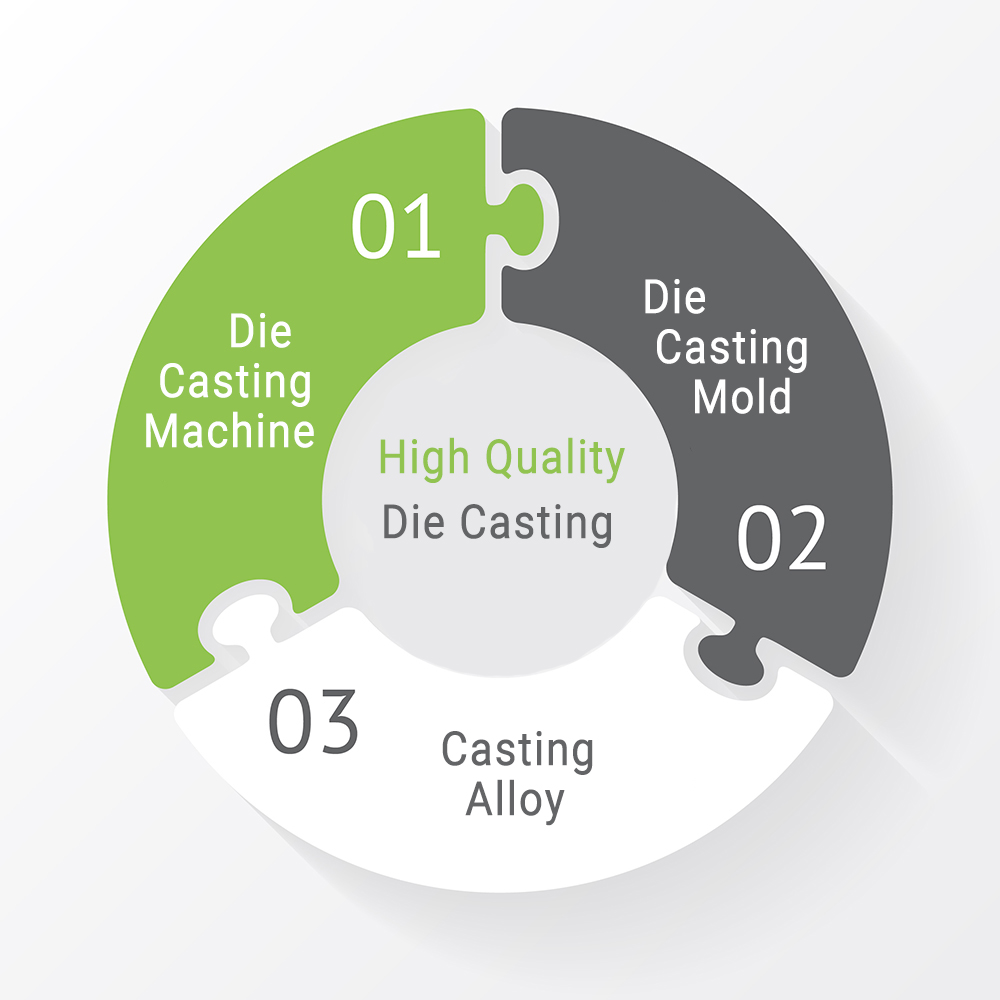Usually, we choose the appropriate casting conditions according to the casting information. Then, reasonable process parameters are calculated from the casting conditions.

• Product information: casting material, weight, quality requirements, projected area;
• Casting conditions: casting pressure, filling speed, filling time
• Process parameters: die casting machine tonnage, punch size, high speed, low speed, gate area

Today we mainly discuss the factors of force in die casting. Mainly include clamping force, injection force, casting pressure, and accumulator pressure. For example, the common data include:

• Accumulator pressure=10Mpa
• Injection force=276KN
• Casting pressure=90Mpa (900bar)
• Clamping force=1600KN(160Ton)

KN, T are units of force, while Mpa, Bar are units of pressure.

## 3.Casting Pressure

The pressure in the die casting process is generated by the injection mechanism of the die casting machine. First, the injection mechanism transmits the pressure to the injection piston through the hydraulic oil. Then the injection piston applies the pressure to the molten metal in the pressure chamber through the injection shot.

The pressure acting on the molten metal is the main factor for obtaining dense and well-defined castings.

The problem is complicated if both the injection force and the injection pressure are considered. Since the injection pressure can better reflect the characteristics of each stage in the die casting process, the casting pressure usually refers to the injection pressure.

##### Injection force

It refers to the force that the hydraulic oil in the injection cylinder of the die casting machine acts on the injection punch to push the molten metal to fill the mold cavity. Its size varies with the specifications of the die-casting machine, reflecting the power of the die-casting machine.

##### Injection specific pressure

It is the static pressure of the molten metal per unit area in the pressure chamber during the injection process. It is proportional to the injection force and inversely proportional to the cross-sectional area of ​​the injection punch.

##### The figure is a typical injection system structure diagram of a die casting machine.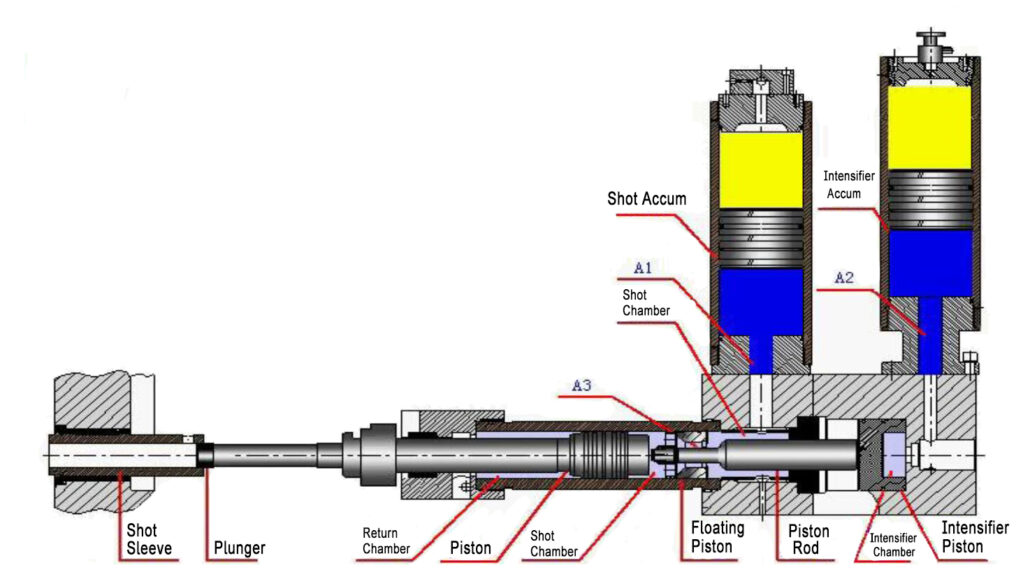The die-casting machine has two accumulators, the left is the fast injection accumulator, and the right is the intensifier accumulator. At the beginning of the slow stage, the injection punch (plunger) is driven by the hydraulic oil to slowly push the molten metal to fill the pressure chamber gradually. The next step is the rapid injection stage, as shown below.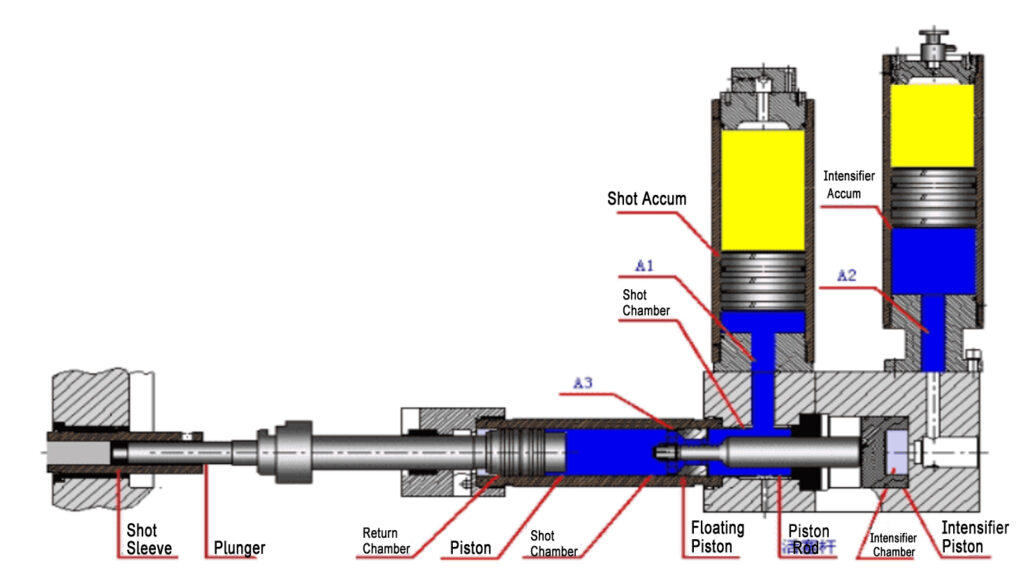In the fast stage, the hydraulic oil in the fast injection accumulator enters the pressure chamber cavity and pushes the punch injection punch to move rapidly. After the molten metal fills the mold, the hydraulic oil in the boost accumulator provides the final intensifier pressure. The casting solidifies under the action of intensifier pressure, as shown in the figure below.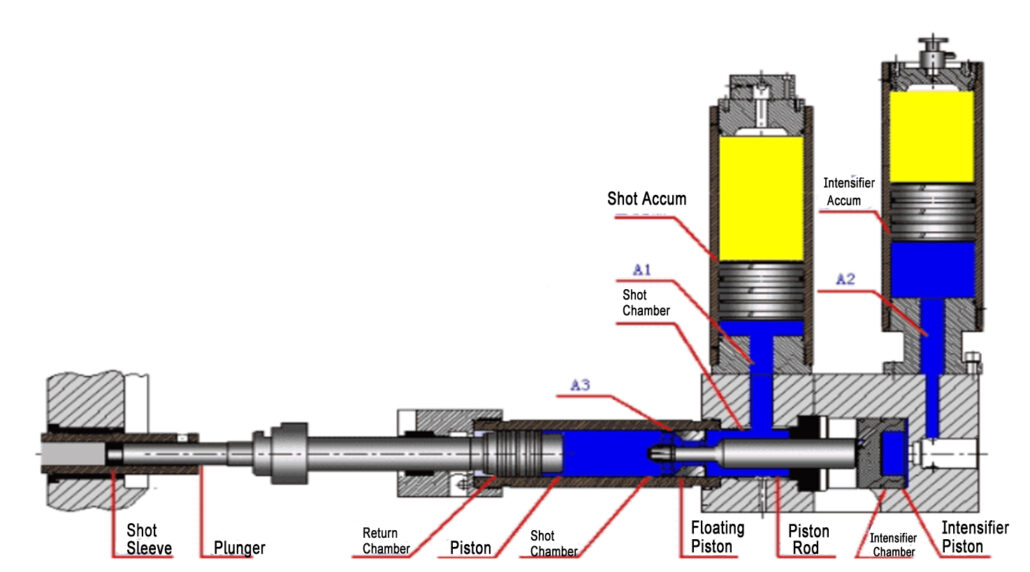The structure of the accumulator is shown in Fig.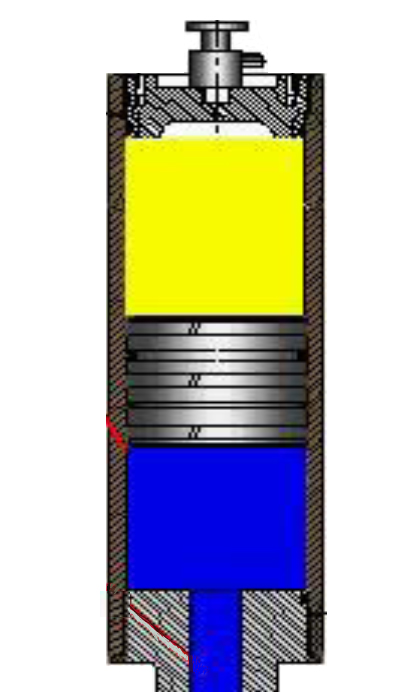The yellow color represents nitrogen gas, and the blue color represents hydraulic oil. In the middle is the piston. Compressed nitrogen provides energy, which exerts pressure on the hydraulic oil through the piston. The hydraulic oil transmits the pressure to the punch through the injection cylinder.

F (force of nitrogen on piston) = F (hydraulic oil on piston)

So P (nitrogen to piston) x S (piston sectional area) = P (hydraulic oil to piston) x S (piston sectional area)

So P (nitrogen) = P (hydraulic oil)

The picture shows the injection punch and injection cylinder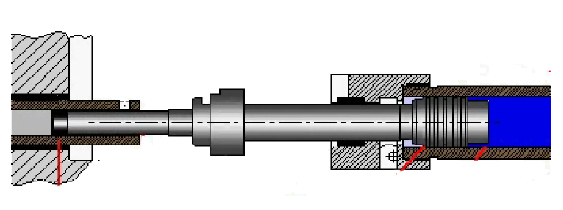##### Force acting on the punch

F (Shot) = F (piston), which can be deduced:

P(Shot) x S(Punch cross-sectional area)=P(Nitrogen) x S(Injection cylinder piston sectional area)

The pressure P of nitrogen is the pressure in the accumulator

So: P(Shot)=S(Punch cross-sectional area)/S(Injection cylinder piston sectional area) x P(Accumulator)

The pressure that the punch acts on the molten metal is equal to the pressure received by the inner cavity of the mold. So the casting pressure P is P (Shot), which is also equal to the boost pressure.#### The relationship between accumulator, casting pressure, and injection force: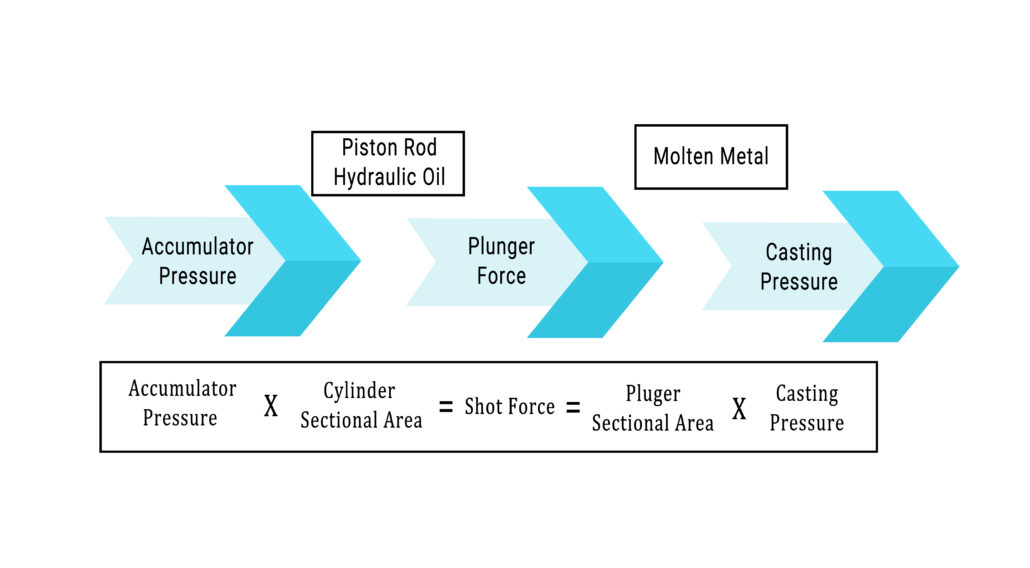Casting pressure summary:

• Conceptually, it belongs to pressure, and the unit is Mpa.
• In space terms, the pressure in the cavity.
• In terms of time, when the last boost is the maximum pressure.
• Numerically equivalent to the maximum boost pressure.

The actual casting pressure will be lost, and the common loss points are:

• During the release of accumulator pressure, the pressure will drop. Loss is 10%.
• The thickness of the biscuit, if the biscuit is too thin, the pressure transmission will be insufficient, and the specific gravity of the casting will decrease.
• The casting temperature is not uniform, resulting in different local casting pressures. According to the analysis of the influence of pressure transfer by the shape of the alloy liquid: the temperature of the alloy liquid is different, the solid-liquid ratio is inconsistent, and the pressure transfer capability is also different.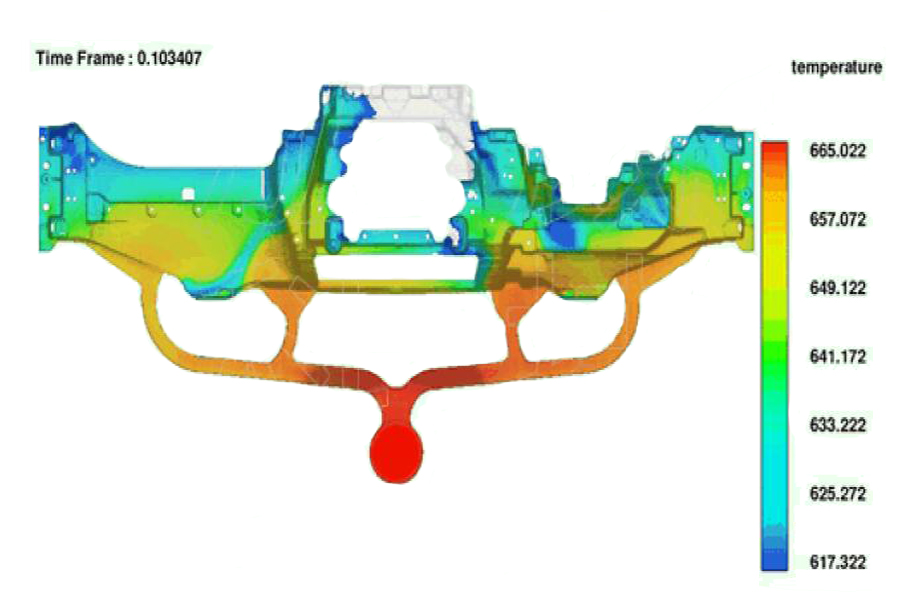## 4.Clamping Force

The picture shows a common integral casting, a1 is the biscuit, a2 is the runner, a3 is the product, and a4 is the overflow.

Casting area A1=a1+a2+a3+a4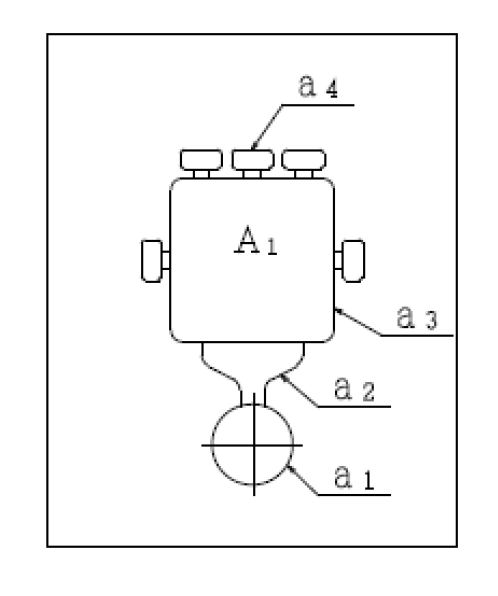During the pressurization stage, the casting has the force to expand the mold, which is called the expansion force F. Usually, due to pressure loss, the bulging force of each part of the die casting is calculated as follows:

F = casting pressure x a3x75% + casting pressure x a4 x 25% + casting pressure x (a1+a2) x 100%

Clamping force refers to the clamping force applied by the die casting machine to the mold to overcome the expansion force of the molten metal on the mold during die casting. What we usually call a 280-ton die-casting machine and a 500-ton die-casting machine, this tonnage is the locking force of the die-casting machine. And the locking force should be greater than 1.1 times the bulging force.

F (clamping force) = 1.1 x F (bulging force) = 1.1 x P (casting pressure) x S (projected area)

In the next section, we will analyze and determine the die casting parameters through a case.